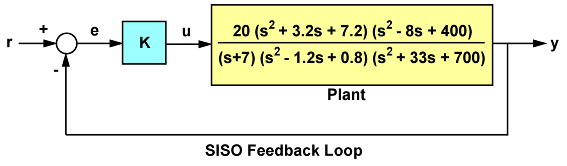# mag2db

Convert magnitude to decibels (dB)

## Syntax

``ydb = mag2db(y)``

## Description

example

````ydb = mag2db(y)` expresses in decibels (dB) the magnitude measurements specified in `y`. The relationship between magnitude and decibels is $ydb=20\ast {\mathrm{log}}_{10}\left(y\right)$```

## Examples

collapse all

For this example, consider the following SISO feedback loop where the system contains multiple gain crossover or phase crossover frequencies, which leads to multiple gain or phase margin values:Create the transfer function.

`G = tf(20,[1 7]) * tf([1 3.2 7.2],[1 -1.2 0.8]) * tf([1 -8 400],[1 33 700]);`

Use the `allmargin` command to compute all stability margins.

`m = allmargin(G)`
```m = struct with fields: GainMargin: [0.3408 3.3920] GMFrequency: [1.9421 16.4807] PhaseMargin: 68.1140 PMFrequency: 7.0776 DelayMargin: 0.1680 DMFrequency: 7.0776 Stable: 1 ```

Note that gain margins are expressed as gain ratios and not in decibels (dB). Use `mag2db` to convert the values to dB.

`GainMargins_dB = mag2db(m.GainMargin)`
```GainMargins_dB = 1×2 -9.3510 10.6091 ```

## Input Arguments

collapse all

Input array, specified as a scalar, vector, matrix, or an array. When `y` is nonscalar, `mag2db` is an element-wise operation.

Data Types: `single` | `double`
Complex Number Support: Yes

## Output Arguments

collapse all

Magnitude measurements in decibels, returned as a scalar, vector, matrix, or an array of the same size as `y`.